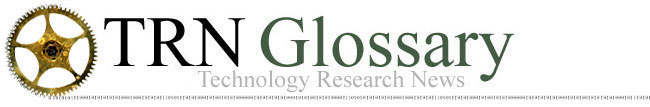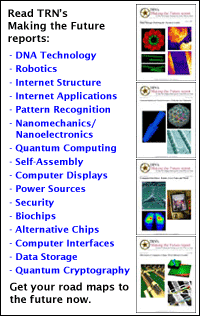A   B   C   D   E   F   G   H   I   J   K   L   M   N   O   P   Q   R   S   T   U   V   W   X   Y   Z

Logic: Methods of reasoning. Logic is the set of principles by which algorithms operate. Boolean Logic is a basic type of logic used in computing.

Logic Gate: Electronic device, typically in integrated circuits, that implements Boolean Logic and uses voltages for true and false values. Logic Gates are the basic building blocks of computer hardware.

A   B   C   D   E   F   G   H   I   J   K   L   M   N   O   P   Q   R   S   T   U   V   W   X   Y   Z